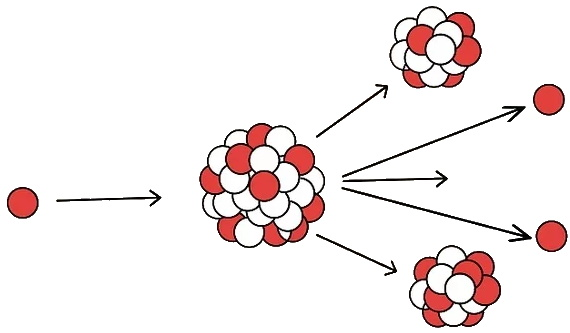#Understand E = mc2

In one of Albert Einstein's revolutionary scientific papers published in 1905, E = mc2 was introduced; where E is energy, m is mass, and c is the speed of light in a vacuum. Since then, E = mc2 has become one of the most famous equations in the world. Even people with no background in physics have at least heard of the equation and are aware of its prodigious influence on the world we live in. However, most people do not exactly know what the equation means. In simple terms, the equation represents the correlation of energy to matter: essentially, energy and matter are but two different forms of the same thing. This relatively simple equation has altered the way we think about energy and provided us with numerous technological advances.

Define the variables of the equation.

The first step to understanding any equation is to know what each variable stands for. In this case, E is the energy of an object at rest, m is the object's mass, and c is the speed of light in vacuum.

The speed of light, c is constant in all reference frames and is roughly equal to 3.00x108 meters per second. In the context of Einstein's relativity, the c2 functions more as a unit conversion factor than a constant. As such, it is squared as a result of dimensional analysis - energy is measured in joules, or kg m2 s-2, so adding the c2 ensures that the relationship between energy and mass is dimensionally consistent.

Understand what is meant by energy.

There are many forms of energy including thermal, electrical, chemical, nuclear and more. Energy is transferred between systems giving power to one system while taking it away from another.

Energy can be neither created nor destroyed, it can only take a different form. For example, coal has a lot of potential energy that turns into thermal energy when it is burned.

Define what mass means.

Mass is generally defined as the amount of matter in an object.

There are also a few other definitions of mass. There exists "invariant mass," and "relativistic mass." Invariant mass is mass that remains unchanged no matter what frame of reference you are in. Relativistic mass, on the other hand, depends on the object's velocity. In the equation E = mc2, m refers to the invariant mass. This is very important, because this means that your mass does not grow as you go faster, contrary to popular belief.

It's important to understand that mass and weight are different. Weight is the gravitational force felt by an object, while mass is the amount of matter in that object. Mass can only change if the object is physically altered, while weight changes depending on the gravity of the environment the object is in. Mass is measured in kilograms (kg) while weight is measured in newtons (N).

Like energy, mass can neither be created nor destroyed, but it can also change form. For example, an ice cube can melt into a liquid, but it still has the same mass in both states.

Realize that mass and energy are equivalent.

The equation states that mass and energy are the same thing and tells you how much energy is contained inside a certain amount of mass. Essentially, the equation explains that a small amount of mass is full of a large amount of energy.

Understand where usable energy comes from.

Most of our consumable energy comes from the burning of coal and natural gas. Burning these substances takes advantage of their valence electrons (unpaired electrons in the outermost shell of an atom) and the bonds they make with other elements. When heat is added, these bonds break and the energy released is used to power our communities.

Obtaining energy this way is not very efficient and is costly to the environment.Apply Einstein's equation to make energy conversion more efficient.

E = mc2 tells us that there is much more energy stored inside the nucleus of an atom than in its valence electrons. The energy released from splitting an atom is much higher than that of breaking electron bonds.

Nuclear power is based on this principle. Nuclear reactors cause fission (the splitting of atoms) to occur and capture the massive amount of energy released.

Discover the technologies made possible by E = mc2.

E = mc2 has enabled the creation of many new and exciting technologies, some of which, we can't imagine living without:

PET scans use radioactivity to see inside the body.
The equation allowed for the development of telecommunications with satellites and rovers.
Radiocarbon dating uses radioactive decay based on the equation to determine the age of ancient objects.
Nuclear energy provides cleaner and more efficient energy sources to our society.

Article source: wikiHow wikiHow is a group effort to create a great resource: the world's largest free how to manual. wikiHow articles help people solve their everyday problems. wikiHow licenses all content under a Creative Commons License. The license allows wikiHow content to be used freely for noncommercial purposes. The Creative Commons License also allows for the creation of derivative works.